Function Repository Resource:

# PolynomialTotalDegree

Get the total degree of a polynomial in any number of variables

Contributed by: Wolfram Staff
 ResourceFunction["PolynomialTotalDegree"][f,vars] gives the total degree of f in the variables vars.

## Examples

### Basic Examples (3)

The term of highest degree is x2y with total degree 3:

 In:=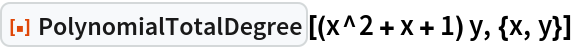Out=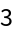Since xSin[x] is not a polynomial, the input here is returned unevaluated:

 In:=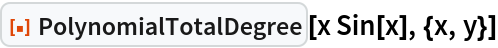Out=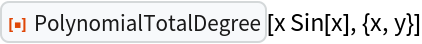This works, though:

 In:=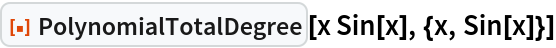Out=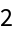George Beck

## Version History

• 1.0.0 – 04 March 2020